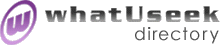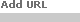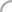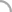SearchThe Directory The Webfor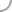Mathematical_PhysicsNew! Submit a site

Categories:Institutions (4) Courses (8)Differential Equations *(100)People (19) Events (30)Topology *(167)

whatUseek Collection Sites (submit a site ):

Give your site great placement in this category in as little as two business days!

whatUseek Directory Site Listings:

An Introduction to Noncommutative Geometry - A set of lecture notes on noncommutative geometry and the applications in physics.

Arrangements and Mathematical Physics - NSF-CBMS Regional Research Conference. January 15 - 19, 2002; Louisiana State University, Baton Rouge.

Burger's Equation - An applet demonstrating Burger's equation in one dimension.

Burger's Equation - An applet demonstrating Burger's equation in one dimension.

Celestial mechanics research - Links, animations, references, and a brief description of research into n-body problems.

Clyde Davenport's Home Page - Summarizes the commutative hypercomplex 4-D mathematics and applies it to electromagnetic theory and special relativity.

Differential Equations and Oscillations - Many problems in physics are described by differential equations. As a complete discussion of differential equations is beyond the scope of this chapter we will deal only with linear first and second order ordinary differential equations.

Dimensional Analysis - A simple review of the powerful technique of dimensional analysis.

Doing Physics with Quaternions - A research effort to see how much of standard physics can be done using only quaternions, a 4-dimensional division algebra.

Five Lectures on Soliton Equations - This is a self-contained review of a new approach to soliton equations of KdV type.

Geometric Algebra and it Applications in Mathematical Physics - A thesis (downloadable in postscript format) on applications of geometric algebra and Clifford algebras in mathematical physics.

Holomorphic Methods in Mathematical Physics - This set of lecture notes gives an introduction to holomorphic function spaces as used in mathematical physics. The emphasis is on the Segal-Bargmann space and the canonical commutation relations.

Homological Methods in Mathematical Physics - These lecture notes are a systematic and self-contained exposition of the cohomological theories naturally related to partial differential equations

Kinetic Theory and its Applications. - This resource is devoted to mathematical aspects of kinetic theory and its applications in physics and chemistry.

Kinetic Theory. Problems and Applications. - This resource is devoted to mathematical aspects of kinetic theory and its applications in physics, chemistry and economics.

Open problems in mathematics and physics - Links to open problems in mathematics a nd physics

Physics of Nonequilibrium Systems Laboratory - The subsidiary of the Department of Air Dynamics and Cosmic Research of Moscow Institute of Physics and Technology. The laboratory deals with the different types of nonequilibrium processes and systems.

Preparation for Gauge Theory - Class lecture notes at a beginning graduate level on the mathematical background needed to understand classical gauge theory.

QMath-8 - Mathematical Results in Quantum Mechanics Symposium to be held in Taxco, Mexico during December 2001.

Radial Symmetric Fourier Transforms - Fourier transforms of radially-symmetric functions can be performed efficiently using the Hankel transform of order zero. Illustrations of the method are presented, and of the Gibbs' phenomenon.

[ 1 2 ]Help build the largest human-edited directory on the web.About   Help   Content Filter   Terms   Privacy Policy© 2018 whatUseek